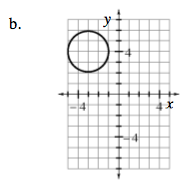Home > A2C > Chapter 7 > Lesson 7.2.3 > Problem7-134

7-134.
1. Write the equation of each circle graphed below. Homework Help ✎

1.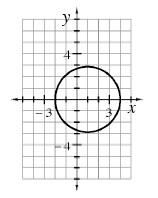2.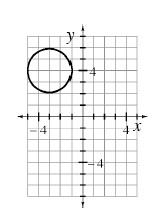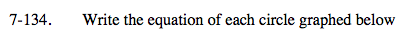Recall the general equation of a circle: (xh)² + (yk)² = r² where (h, k) is the center of the circle and r is the radius.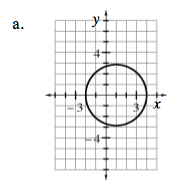Write in graphing form:
(x − 1)2 + y2 = 9